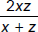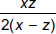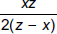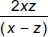# User ForumSubject :IMO    Class : Class 8

If ax = by = cz and b2 = ac, then y equals

ABCDI kinda need help with this sum, i use the formula a^x = b^y = c^z = k, but is there any other simple way to do this particular sum? I have done sums like these before but this one is a little tricky